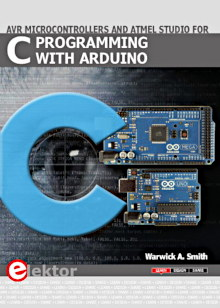Starting Electronics needs your help! Please make a donation to help cover our hosting and other costs. Click the donate button to send a donation of any amount.# Tutorial 8: LED Knight Rider Display in VHDL

Created on: 9 January 2013

A LED chaser type knight rider display with 8 LEDs written in VHDL and implemented on a CPLD.

This video shows the knight-rider display on the home built CPLD board.

Can't see the video? View on YouTube →

## Knight Rider VHDL Code

The code listing for the LED knight rider display is shown below.

```library IEEE;
use IEEE.STD_LOGIC_1164.ALL;
use IEEE.STD_LOGIC_UNSIGNED.ALL;

entity knight_top is
Port ( CLK : in  STD_LOGIC;
LED : out  STD_LOGIC_VECTOR (7 downto 0));
end knight_top;

architecture Behavioral of knight_top is

signal clk_div   : std_logic_vector(4 downto 0);
signal shift_reg : std_logic_vector(7 downto 0) := X"01";
signal fwd       : std_logic := '1';

begin

-- clock divider
process (CLK)
begin
if (CLK'Event and CLK = '1') then
clk_div <= clk_div + '1';
end if;
end process;

-- knight rider display
process (clk_div(4))
begin
if (clk_div(4)'Event and clk_div(4) = '1') then
if (fwd = '1') then
shift_reg <= shift_reg(6 downto 0) & '0';
if (shift_reg = X"40") then
fwd <= '0';
end if;
else
shift_reg <= '0' & shift_reg(7 downto 1);
if (shift_reg = X"02") then
fwd <= '1';
end if;
end if;
end if;
end process;

-- display the result on the LEDs
LED <= not shift_reg;

end Behavioral;
```

### Source Code

The above VHDL source code with the UCF and JED files can be downloaded here: knight-rider-VHDL.zip (6.3kB)

### Clock Divider

A clock divider is is used to slow down the input clock and make the knight rider display slow enough to see. The input clock is a 130Hz clock from the AVR on the board (see tutorial 6 for information on how to set the clock up).

### Initializing Registers

In the line of code signal shift_reg : std_logic_vector(7 downto 0) := X"01"; the 8-bit register shift_reg is initialized to a hexadecimal value of 01. The 1 represents the LED that will be on in the display.

The value is assigned to the register using the VHDL := operator and is declared to be a hexadecimal number by preceding the number with X.

The fwd signal is also assigned a default value which is a single bit value of 1.

### Knight Rider Process

The knight rider process is driven by the rising edge of the divided clock pulse. The shift register shift_reg contains 0x01 initially and the fwd signal indicates that the display is moving in the forward direction (i.e. shifting from right to left).

On each clock pulse, the value in shift_reg is shifted left once until the upper bit in shift_reg contains the high bit (logic 1 bit). The fwd signal flag will then be changed to a value of 0, indicating that the direction of shifting will be reversed (i.e. start shifting from left to right).

The fwd flag is checked in the VHDL if-then-else-endif construct. If fwd contains 1, the code under the if statement will run. If fwd contains 0, the code under the else statement will run.

When fwd is cleared, shifting takes place from left to right until the high bit has been moved to the first bit position in shift_reg. fwd will then be set to 1 and the whole process starts again.

### LED Outputs

The code LED <= not shift_reg; displays the value of the shift register on the LEDs. It inverts the value in the shift register using the VHDL not keyword to compensate for the inverting LEDs on the home built CPLD board.

Books that may interest you: